Question

# Consider the following reaction at 298 K. C(graphite)+2H2(g)⟶CH4(g)ΔH∘=−74.6 kJ and ΔS∘=−80.8 J/KC(graphite)+2H2(g)⟶CH4(g)ΔH∘=−74.6 kJ and ΔS∘=−80.8 J/K...

Consider the following reaction at 298 K.

C(graphite)+2H2(g)⟶CH4(g)ΔH∘=−74.6 kJ and ΔS∘=−80.8 J/KC(graphite)+2H2(g)⟶CH4(g)ΔH∘=−74.6 kJ and ΔS∘=−80.8 J/K

Calculate the following quantities.

ΔSsys=ΔSsys

J/K

ΔSsurr=

J/K

ΔSuniv=

J/K

C(graphite) + 2H2(g)⟶CH4(g) ΔHo = −74.6 kJ and ΔSo= −80.8 J/K

ΔSsys = ΔSo

ΔSsys = − 80.8 J/K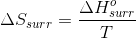ΔHosurr = − ΔHo

ΔHosurr = 74.6 kJ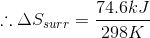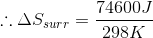ΔSsurr = 250.3 J/K

ΔSuniv = ΔSsys + ΔSsurr

ΔSuniv = − 80.8 J/K + 250.3 J/K

ΔSuniv = 169.5 J/K

#### Earn Coins

Coins can be redeemed for fabulous gifts.

Similar Homework Help Questions
• ### Consider the reaction at 298 K. C(graphite)+2 H 2 (g)⟶ CH 4 (g)Δ?°=−74.6 kJ C(graphite)+2H2(g)⟶CH4(g)ΔH°=−74.6 kJ...

Consider the reaction at 298 K. C(graphite)+2 H 2 (g)⟶ CH 4 (g)Δ?°=−74.6 kJ C(graphite)+2H2(g)⟶CH4(g)ΔH°=−74.6 kJ Calculate the quantities. Delta Ssys=_______ J/K Delata Ssurr =_______ J/K

• ### Consider the following reaction at 298 K. C(graphite) + 2H2(g)→ CH4(g) ΔH°=-74.6 kJ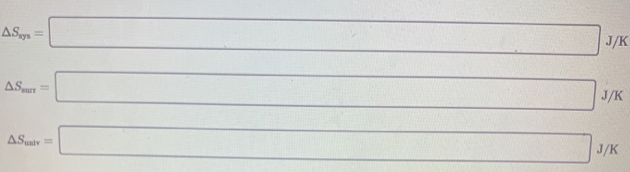Consider the following reaction at 298 K.C(graphite) + 2H2(g)→ CH4(g)  ΔH°=-74.6 kJ

• ### Consider the following reaction at 298 K. AH' - -74.6 kJ and AS C(graphite) + 2...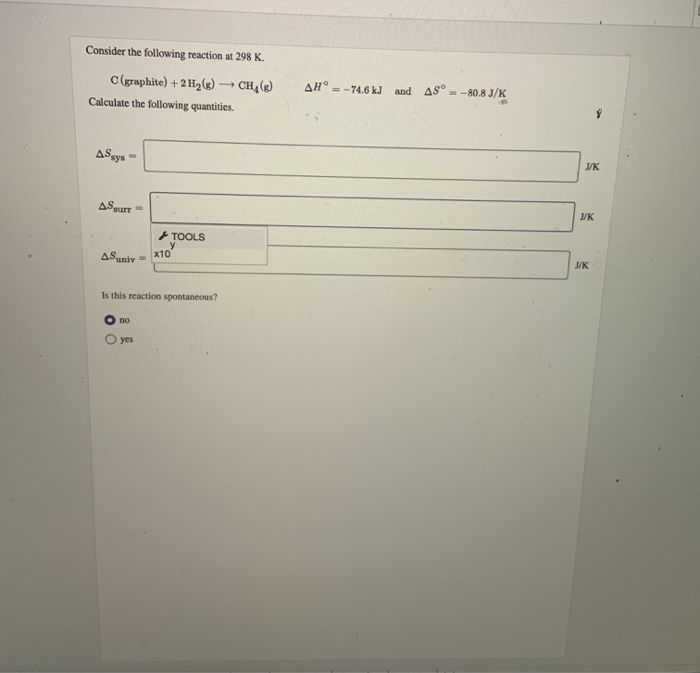Consider the following reaction at 298 K. AH' - -74.6 kJ and AS C(graphite) + 2 H2(g) - CH (8) Calculate the following quantities. -80.8 J/K ASxys - + TOOLS AS univ- X10 Is this reaction spontaneous? Ово O yes

• ### Consider the following reaction at 298 K. C(graphite) + 2 H,(8) CH () AH° = -74.6...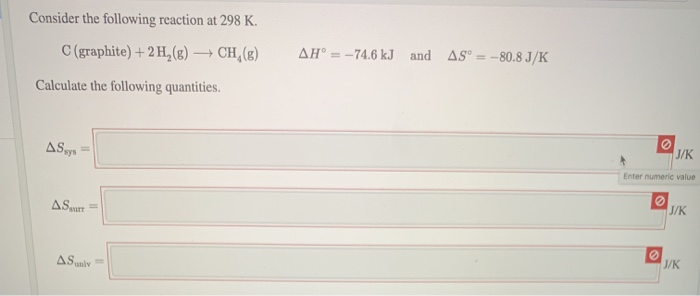Consider the following reaction at 298 K. C(graphite) + 2 H,(8) CH () AH° = -74.6 kJ and AS° = -80.8 J/K Calculate the following quantities. Enter numeric value

• ### Consider the following reaction at 298 K. C(graphite)+2Cl2(g)⟶CCl4(l)ΔH∘=−139 kJC(graphite)+2Cl2(g)⟶CCl4(l)ΔH∘=−139 kJ Calculate the following quantities. Refer to...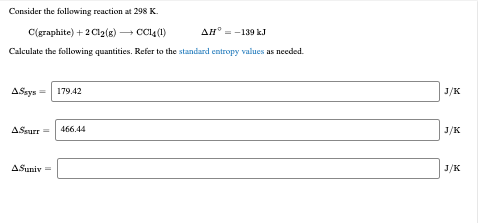Consider the following reaction at 298 K. C(graphite)+2Cl2(g)⟶CCl4(l)ΔH∘=−139 kJC(graphite)+2Cl2(g)⟶CCl4(l)ΔH∘=−139 kJ Calculate the following quantities. Refer to the standard entropy values as needed. Consider the following reaction at 298 K. C(graphite) + 2Cl2(g) C014 (1) AH = -139 kJ Calculate the following quantities. Refer to the standard entropy values as needed. A\$sys = 179.42 ASsurr = 466.44 A.Sunix =

• ### 1a) Consider the following reaction: 3 C(s) + 4 H2(g) → C3H8(g); ΔH° = –104.7 kJ;...

1a) Consider the following reaction: 3 C(s) + 4 H2(g) → C3H8(g); ΔH° = –104.7 kJ; ΔS° = –287.4 J/K at 298 K What is the equilibrium constant at 298 K for this reaction? Report answer to TWO significant figures. 1b) Τhe enthalpy of vaporization of ammonia is 23.35 kJ/mol at its boiling point (–33 °C). Calculate the value of ΔSsurr when 1.00 mole of ammonia is vaporized at –33 °C and 1.00 atm. Report answer to THREE significant figures.

• ### For the following reaction, N2 (g) + 1/2O2 (g)  N2O (g) At 298 K, ΔSsurr = -...

For the following reaction, N2 (g) + 1/2O2 (g)  N2O (g) At 298 K, ΔSsurr = - 275.34 J/K and  ΔSsys = -74.3 J/K. Which of the following statements is true? ΔSuniv = -201.04 J/K and the process is nonspontaneous at 298K ΔSuniv = -201.04 J/K and the process is spontaneous at 298K ΔSuniv = 201.04 J/K and the process is spontaneous at 298K ΔSuniv = -349.6 J/K and the process is nonspontaneous at 298K ΔSuniv = -349.6 J/K and the process...

• ### A reaction has a ΔH°= 204.6 kJ and a ΔS°= -19.5 J/K. a. Given the sign...

A reaction has a ΔH°= 204.6 kJ and a ΔS°= -19.5 J/K. a. Given the sign and magnitude of ΔH and ΔS, will the reaction be spontaneous at all temperatures, nonspontaneous at all temperatures? Briefly explain your reasoning. b. Calculate the standard free energy change for the reaction at 298 K. c. Is the reaction spontaneous or nonspontaneous at 298 K?

• ### ​ Consider the following reaction at 298 K: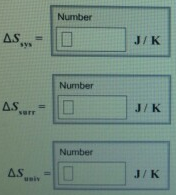Consider the following reaction at 298 K: C(graphite) + 2CI2(g)→ CCI4(l)  ΔH°=-139 kJ Calculate the following quantities.

• ### Calculate Δ Hrxn for the following reaction: CH4(g)+4Cl2(g)→CCl4(g)+4HCl(g) given these reactions and their ΔH values: C(s)C(s)H2(g)+++2H2(g)2Cl2(g)Cl2(g)→→→CH4(g),CCl4(g),2HCl(g),ΔH=−74.6...

Calculate Δ Hrxn for the following reaction: CH4(g)+4Cl2(g)→CCl4(g)+4HCl(g) given these reactions and their ΔH values: C(s)C(s)H2(g)+++2H2(g)2Cl2(g)Cl2(g)→→→CH4(g),CCl4(g),2HCl(g),ΔH=−74.6 kJΔH=−95.7 kJΔH=−184.6 kJ﻿